In :
from IPython.display import Image
Image("images/F2.png",width=300)

Out:In :
Image("images/dieti.png",width=200)

Out:# Introduction to Quantum Circuits¶

Tutorial on the 1 Q-bit gates in Qiskit

In :
Image("images/Qiskit.png",width=100)

Out:Qiskit is an open-source framework for quantum computing. It provides tools for creating and manipulating quantum programs and running them on prototype quantum devices on IBM Q Experience or on simulators on a local computer. It follows the circuit model for universal quantum computation, and can be used for any quantum hardware (currently supports superconducting qubits and trapped ions) that follows this model.

In :
from qiskit import *
from qiskit.visualization import plot_histogram, plot_bloch_vector, plot_bloch_multivector, circuit_drawer
from qiskit_textbook.widgets import plot_bloch_vector_spherical
from math import sqrt, pi
from IPython.display import display, Math, Latex

In :
n=1
qc = QuantumCircuit(n)  # Create a quantum circuit with one qubit
backend = Aer.get_backend('statevector_simulator') # Tell Qiskit how to simulate our circuit

$$| \psi_{in}> = \frac{\sqrt{3}}{2} |0 \rangle + \frac{j}{2} |1 \rangle$$
In :
initial_state = [sqrt(3)/2,1j/2]  # Define state |q_0>
print(initial_state)
plot_bloch_multivector(initial_state)

[0.8660254037844386, 0.5j]

Out: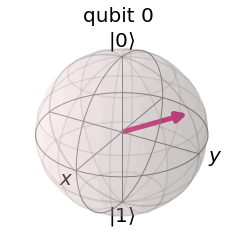In :
qc.initialize(initial_state, 0) # Apply initialisation operation to the 0th qubit
qc.draw(output='mpl') # Draw the corresponding circuit

Out: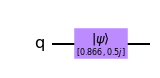We now apply the not (X-) gate:

$$X = \begin{pmatrix} 0 & 1 \\ 1 & 0 \end{pmatrix}$$
In :
qc.x(0) #Apply X-gate
qc.draw(output='mpl') # Draw the corresponding circuit

Out: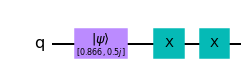In :
result = execute(qc,backend).result() # Do the simulation, returning the result
out_state = result.get_statevector() # Get the final statevector of an experiment.
print(out_state)
plot_bloch_multivector(out_state)

[0.       +0.5j 0.8660254+0.j ]

Out: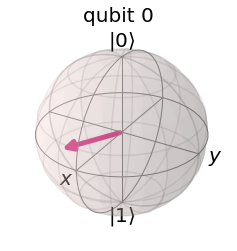In :
results = result.get_counts() # Get the histogram data of an experiment.
print(out_state) # Display the output state vector

[0.       +0.5j 0.8660254+0.j ]

$$\begin{pmatrix} 0 & 1 \\ 1 & 0 \end{pmatrix} \left( \begin{array}{c} \frac{\sqrt{3}}{2} \\ \frac{j}{2} \end{array} \right)$$
$$| \psi_{out}> = \frac{j}{2} |0> + \frac{\sqrt{3}}{2} |1>$$
In :
plot_histogram(results) # Display the output state vector

Out: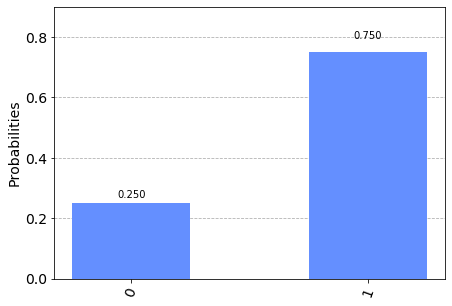# Bibliography¶

Qiskit: An Open-source Framework for Quantum Computing (2019), doi:10.5281/zenodo.2562110

N. David Mermin "Quantum Computer Science (An Introduction)" (2007)